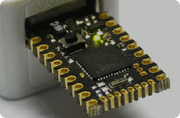# Flashing Lights

This Tutorial assumes that you are able to enter code in Espruino. If you can't do that yet, please follow the steps on the Quick Start page.

## Introduction

If you've followed the Quick Start, you've already made an LED flash. In this tutorial, we'll show some other ways of flashing lights.

## Wiring Up

We're just going to use the LEDs on the board for this. If you want to drive something bigger (like a chain of christmas tree lights), you'll have to make a small circuit.

As time goes on we will cover this in more detail, so stay tuned!

## Software

### Try 1

The example in the Quick Start shows how to flash an LED the 'right' way - but we can actually do it in one simple line. Just copy and paste:

``````var l;setInterval("digitalWrite(LED1,l=!l);",200);
``````

The function setInterval calls the first argument (either a function or a string containing code to execute) every 200ms. In this case, the code just flips the state of a variable `l` (which we had to define first with `var l`) between `true` and `false` with `l=!l`, and sets the state of the LED to it. This isn't recommended as it's a bit hard to read though.

To stop the light flashing, just type `clearInterval()`

### Try 2

Try 1 shows how to flash LEDs at a set rate. But what if you want to flash two LEDs at two different time periods?

This time, we're going to write the code in a way that's easier to understand. First off, write two functions:

``````var on1,on2;
function toggle1() {
on1 = !on1;
digitalWrite(LED1, on1);
}
function toggle2() {
on2 = !on2;
digitalWrite(LED2, on2);
}
``````

Note that each function uses its own variable to keep track of whether the LED should be on or off.

Now, you can use setInterval twice, one for each function. And each time you can give it a different time period:

``````setInterval(toggle1, 400);
setInterval(toggle2, 456);
``````

This produces a nice effect as the lights move in and out of phase. To add a third light, just create a third function and call `setInterval()` again.

Each time you called `setInterval()`, it returned a different number. If you want to change how fast the interval runs (or cancel it altogether) you need to use this number:

``````changeInterval(1,1000);
``````

or

``````clearInterval(1);
``````

### Try 3

But this is less easy than it could be. In JavaScript, you can define variables inside functions - so we can make one function which stores the 'on' variable for each LED, and which starts the timer as well!

First, reset Espruino so everything goes back to the way it was:

``````reset()
``````

Then, create the function:

``````function startFlashing(pin, period) {
var on = false;
setInterval(function() {
on = !on;
digitalWrite(pin, on);
}, period);
}
``````

Now we can call this function for however many LEDs we want:

``````startFlashing(LED1, 400);
startFlashing(LED2, 456);
``````

### Try 4

Since JavaScript is Object-Oriented, and each LED is an instance of `Pin`, we can do the following as well:

``````Pin.prototype.startFlashing = function(period) {
var on = false;
var pin = this;
setInterval(function() {
on = !on;
digitalWrite(pin, on);
}, period);
}
LED1.startFlashing(100);
``````

Note that, like Numbers and Strings, Pins can not themselves contain any extra values. So if we wanted to be able to call startFlashing multiple times on the same LED we'd have to store the interval's ID somewhere else (like in the Pin object):

``````Pin.prototype.startFlashing = function(period) {
if (Pin.intervals==undefined) Pin.intervals = [];
if (Pin.intervals[this]) clearInterval(Pin.intervals[this]);
var on = false;
var pin = this;
Pin.intervals[this] = setInterval(function() {
on = !on;
digitalWrite(pin, on);
}, period);
}
``````

Now, you can call `startFlashing` multiple times:

``````LED1.startFlashing(10);
LED1.startFlashing(100);
``````

### Try 5

We can also flash in different ways. For instance, every 1/50th of a second we could randomly decide whether we want the LED to be on or off (remember to reset Espruino using the reset() function before trying this - or maybe just do it on another LED if your board has more than 2):

``````setInterval(function() {
digitalWrite(LED1, Math.random()>0.5);
}, 20);
``````

`Math.random()` returns a random number between 0 and 1. By checking if it is above 0.5 or not, we can give the LED a 50/50 chance of being on. In this code, 20 means 20ms. This is 20 1/1000ths of a second - which is 50 times a second.

This page is auto-generated from GitHub. If you see any mistakes or have suggestions, please let us know.